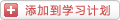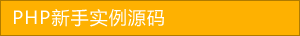﻿ __construct() 和 __destory() 在 PHP 中需要注意的地方 - php高级应用 - PHP粉丝网

# __construct() 和 __destory() 在 PHP 中需要注意的地方

##### 发布:smiling　来源: PHP粉丝网 　添加日期：2022-06-17 11:06:24　浏览: 评论：0

1. class A
2.
3.
4.     public \$name
5.
6.     public function __construct(\$name
7.
8.     {
9.
10.         \$this->name = \$name
11.
12.         echo "A：构造函数被调用，{\$this->name}", PHP_EOL;
13.
14.     }
15.
16.     public function __destruct()
17.
18.     {
19.
20.         echo "A：析构函数被调用，{\$this->name}", PHP_EOL;
21.
22.     }
23.
24.
25. \$a = new A('\$a');
26.
27. echo '-----', PHP_EOL;
28.
29. class B extends A
30.
31.
32.     public function __construct(\$name
33.
34.     {
35.
36.         \$this->name = \$name
37.
38.         parent::__construct(\$name);
39.
40.         echo "B：构造函数被调用，{\$this->name}", PHP_EOL;
41.
42.     }
43.
44.     public function __destruct()
45.
46.     {
47.
48.         parent::__destruct();
49.
50.         echo "B：析构函数被调用，{\$this->name}", PHP_EOL;
51.
52.     }
53.
54.
55. class C extends A
56.
57.
58.     public function __construct(\$name
59.
60.     {
61.
62.         \$this->name = \$name
63.
64.         echo "C：构造函数被调用，{\$this->name}", PHP_EOL;
65.
66.     }
67.
68.     public function __destruct()
69.
70.     {
71.
72.         echo "C：析构函数被调用，{\$this->name}", PHP_EOL;
73.
74.     }
75.
76.
77. class D extends A
78.
79.
80.
81. // unset(\$a); // \$a的析构提前
82.
83. // \$a = null; // \$a的析构提前
84.
85. \$b = new B('\$b');
86.
87. \$c = new C('\$c');
88.
89. \$d = new D('\$d');
90.
91. echo '-----', PHP_EOL;exit
92.
93. // A：构造函数被调用，\$a
94.
95. // -----
96.
97. // A：构造函数被调用，\$b
98.
99. // B：构造函数被调用，\$b
100.
101. // C：构造函数被调用，\$c
102.
103. // A：构造函数被调用，\$d
104.
105. // -----
106.
107. // A：析构函数被调用，\$d
108.
109. // C：析构函数被调用，\$c
110.
111. // A：析构函数被调用，\$b
112.
113. // B：析构函数被调用，\$b
114.
115. // A：析构函数被调用，\$a

1. class E
2.
3.
4.     public \$name
5.
6.     public \$obj
7.
8.     public function __destruct()
9.
10.     {
11.
12.         echo "E：析构函数被调用，" . \$this->name, PHP_EOL;
13.
14.         echo '-----', PHP_EOL;
15.
16.     }
17.
18.
19. \$e1 = new E();
20.
21. \$e1->name = 'e1'
22.
23. \$e2 = new E();
24.
25. \$e2->name = 'e2'
26.
27. \$e1->obj = \$e2
28.
29. \$e2->obj = \$e1

1. \$e1 = new E();
2.
3. \$e1->name = 'e1'
4.
5. \$e2 = new E();
6.
7. \$e2->name = 'e2'
8.
9. \$e1->obj = \$e2
10.
11. \$e2->obj = \$e1
12.
13. \$e1 = null;
14.
15. \$e2 = null;
16.
17. // gc_collect_cycles();
18.
19. \$e3 = new E();
20.
21. \$e3->name = 'e3'
22.
23. \$e4 = new E();
24.
25. \$e4->name = 'e4'
26.
27. \$e3->obj = \$e4
28.
29. \$e4->obj = \$e3
30.
31. \$e3 = null;
32.
33. \$e4 = null;
34.
35. echo 'E destory', PHP_EOL;

1. // 不使用gc回收的结果
2.
3. // E destory
4.
5. // E：析构函数被调用，e1
6.
7. // -----
8.
9. // E：析构函数被调用，e2
10.
11. // -----
12.
13. // E：析构函数被调用，e3
14.
15. // -----
16.
17. // E：析构函数被调用，e4
18.
19. // -----

1. // 使用gc回收后结果
2.
3. // E：析构函数被调用，e1
4.
5. // -----
6.
7. // E：析构函数被调用，e2
8.
9. // -----
10.
11. // E destory
12.
13. // E：析构函数被调用，e3
14.
15. // -----
16.
17. // E：析构函数被调用，e4
18.
19. // -----

1. class F
2.
3.
4.     public function f()
5.
6.     {
7.
8.         // Deprecated: Methods with the same name as their class will not be constructors in a future version of PHP; F has a deprecated constructor
9.
10.         echo "F：这也是构造函数，与类同名，不区分大小写", PHP_EOL;
11.
12.     }
13.
14.     // function F(){
15.
16.     //     // Deprecated: Methods with the same name as their class will not be constructors in a future version of PHP; F has a deprecated constructor
17.
18.     //     echo "F：这也是构造函数，与类同名", PHP_EOL;
19.
20.     // }
21.
22.     // function __construct(){
23.
24.     //     echo "F：这是构造函数，__construct()", PHP_EOL;
25.
26.     // }
27.
28.
29. \$f = new F();

PHP是不运行方法的重载的，只支持重写，就是子类重写父类方法，但不能定义多个同名方法而参数不同。在Java等语言中，重载方法非常方便，特别是在类实例化时，可以方便地实现多态能力。

\$r1 = new R(); // 默认构造函数

\$r2 = new R('arg1'); // 默认构造函数 一个参数的构造函数重载，arg1

\$r3 = new R('arg1', 'arg2'); // 默认构造函数 两个参数的构造函数重载，arg1，arg2

1. class R
2.
3.
4.     private \$a
5.
6.     private \$b
7.
8.     public function __construct()
9.
10.     {
11.
12.         echo '默认构造函数', PHP_EOL;
13.
14.         \$argNums = func_num_args();
15.
16.         \$args = func_get_args();
17.
18.         if (\$argNums == 1) {
19.
20.             \$this->constructA(...\$args);
21.
22.         } elseif (\$argNums == 2) {
23.
24.             \$this->constructB(...\$args);
25.
26.         }
27.
28.     }
29.
30.     public function constructA(\$a
31.
32.     {
33.
34.         echo '一个参数的构造函数重载，' . \$a, PHP_EOL;
35.
36.         \$this->a = \$a
37.
38.     }
39.
40.     public function constructB(\$a\$b
41.
42.     {
43.
44.         echo '两个参数的构造函数重载，' . \$a . '，' . \$b, PHP_EOL;
45.
46.         \$this->a = \$a
47.
48.         \$this->b = \$b
49.
50.     }
51.
52.
53. \$r1 = new R(); // 默认构造函数
54.
55. \$r2 = new R('arg1'); // 默认构造函数 一个参数的构造函数重载，arg1
56.
57. \$r3 = new R('arg1''arg2'); // 默认构造函数 两个参数的构造函数重载，arg1，arg2

1. class Singleton
2.
3.
4.     private static \$instance
5.
6.     public static function getInstance()
7.
8.     {
9.
10.         return self::\$instance == null ? self::\$instance = new Singleton() : self::\$instance
11.
12.     }
13.
14.     private function __construct()
15.
16.     {
17.
18.     }
19.
20.
21. \$s1 = Singleton::getInstance();
22.
23. \$s2 = Singleton::getInstance();
24.
25. echo \$s1 === \$s2 ? 's1 === s2' : 's1 !== s2', PHP_EOL;
26.
27. // \$s3 = new Singleton(); // Fatal error: Uncaught Error: Call to private Singleton::__construct() from invalid context

### 分享到： document.write('<a href="http://v.t.sina.com.cn/share/share.php?url='+encodeURIComponent(location.href)+'&appkey=3172366919&title='+encodeURIComponent('__construct() 和 __destory() 在 PHP 中需要注意的地方')+'" title="分享到新浪微博" class="t1" target="_blank">&nbsp;</a>'); document.write('<a href="http://www.douban.com/recommend/?url='+encodeURIComponent(location.href)+'&title='+encodeURIComponent('__construct() 和 __destory() 在 PHP 中需要注意的地方')+'" title="分享到豆瓣" class="t2" target="_blank">&nbsp;</a>'); document.write('<a href="http://share.renren.com/share/buttonshare.do?link='+encodeURIComponent(location.href)+'&title='+encodeURIComponent('__construct() 和 __destory() 在 PHP 中需要注意的地方')+'" title="分享到人人" class="t3" target="_blank">&nbsp;</a>'); document.write('<a href="http://www.kaixin001.com/repaste/share.php?rtitle='+encodeURIComponent('__construct() 和 __destory() 在 PHP 中需要注意的地方')+'&rurl='+encodeURIComponent(location.href)+'&rcontent=" title="分享到开心网" class="t4" target="_blank">&nbsp;</a>'); document.write('<a href="http://sns.qzone.qq.com/cgi-bin/qzshare/cgi_qzshare_onekey?url='+encodeURIComponent(location.href)+'" title="分享到QQ空间" class="t5" target="_blank">&nbsp;</a>');